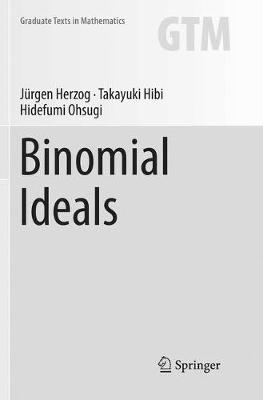•# Binomial Ideals - Graduate Texts in Mathematics 279 (Paperback)

(author), (author), (author)
£44.99
Paperback 321 Pages / Published: 31/01/2019
• We can order this from the publisher

Usually dispatched within 15 working days

This textbook provides an introduction to the combinatorial and statistical aspects of commutative algebra with an emphasis on binomial ideals. In addition to thorough coverage of the basic concepts and theory, it explores current trends, results, and applications of binomial ideals to other areas of mathematics.
The book begins with a brief, self-contained overview of the modern theory of Groebner bases and the necessary algebraic and homological concepts from commutative algebra. Binomials and binomial ideals are then considered in detail, along with a short introduction to convex polytopes. Chapters in the remainder of the text can be read independently and explore specific aspects of the theory of binomial ideals, including edge rings and edge polytopes, join-meet ideals of finite lattices, binomial edge ideals, ideals generated by 2-minors, and binomial ideals arising from statistics. Each chapter concludes with a set of exercises and a list of related topics and results that will complement and offer a better understanding of the material presented.
Binomial Ideals is suitable for graduate students in courses on commutative algebra, algebraic combinatorics, and statistics. Additionally, researchers interested in any of these areas but familiar with only the basic facts of commutative algebra will find it to be a valuable resource.

Publisher: Springer Nature Switzerland AG
ISBN: 9783030070199
Number of pages: 321
Weight: 528 g
Dimensions: 235 x 155 mm
Edition: Softcover reprint of the original 1st ed. 201